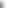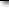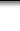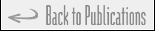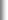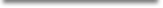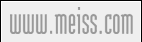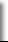# Partitioning Heuristics for the Multi-Item Capacitated Lot Sizing Problem## Awi Federgruen, Jörn Meissner, Michal TzurAbstractThis paper addresses the multi-item capacitated lot sizing problem. We consider a family of $N$ items which are produced in or obtained from the same production facility. Demands are deterministic for each item and each period within a given horizon of $T$ periods.

First, we consider the case that a single setup cost is incurred in each period when an order for any item is placed in that period. We develop an exact branch-and-bound method which can be efficiently used for problems of moderate size. For large problems we propose a partioning heuristic. The partitioning heuristic partitions the complete $T$ periods into smaller intervalls, and specifies an associated dynamic lot sizing model for each of these. The intervals are of a size which permits the use of the exact branch-and-bound method.

The partitioning heuritic can, in the single item case, be implemented with complexity O($T^2 \log \log T$) and for the general multi-item model, in O($N^2 T^2 \log T \log C^*$) times where $C^*$ represents the largest among all periods' capacities. We show that our heuristic is $\epsilon-$optimal as $T\rightarrow\infty$, provided that some of the models paramters are uniformly bounded from above and from below.

Subsequently, we further generalize the model to include additional item dependend set-up costs and provide extensive numerial studies to evaluate the performance under various data constellations.Keywords### supply chain management, inventory models, lot sizing, time partitioningStatusArbeitspapierDownloadRevised and renamed in November 2003: Progressive Interval Heuristics for Multi-Item Capacitated Lot Sizing ProblemsReferenceBibTeX, Plain Text